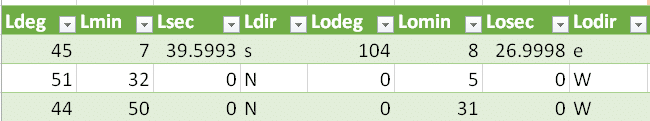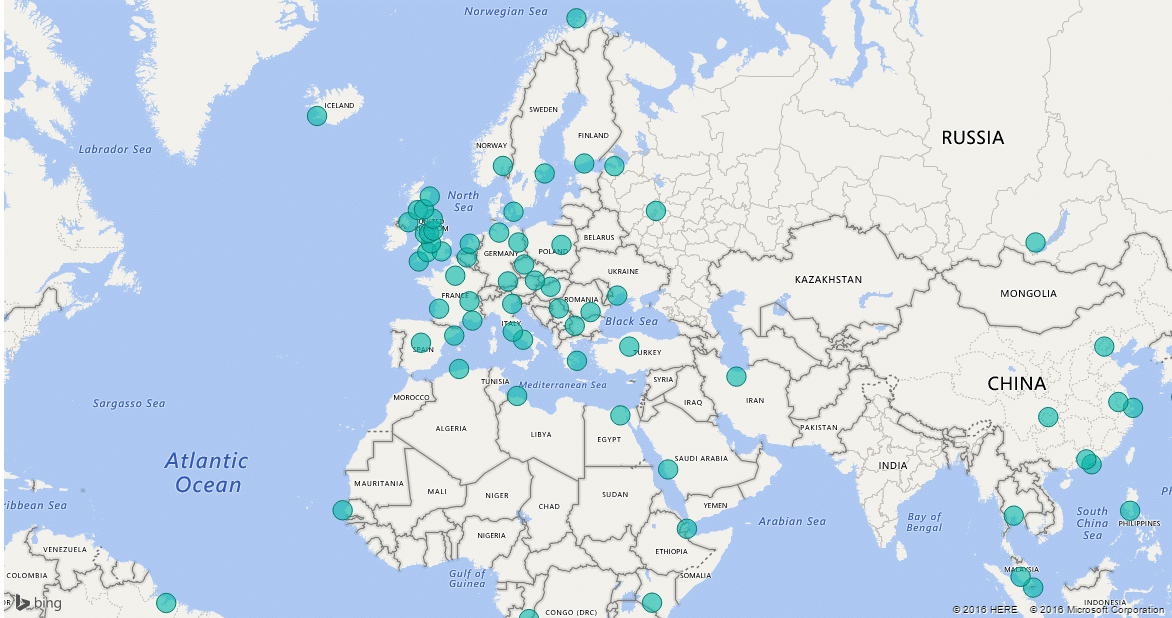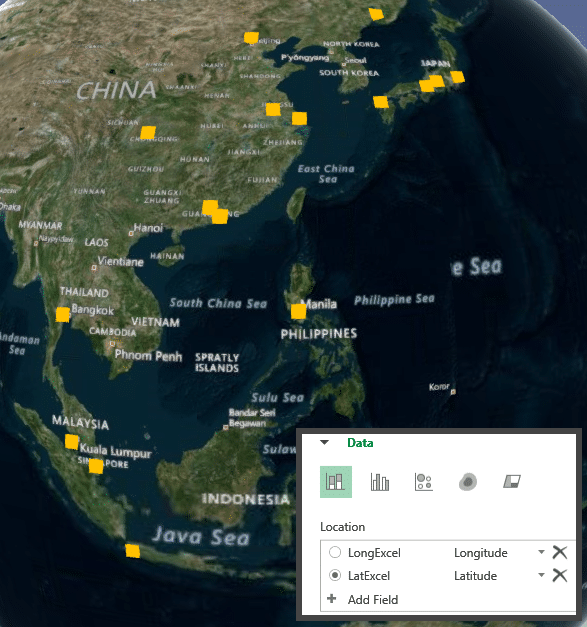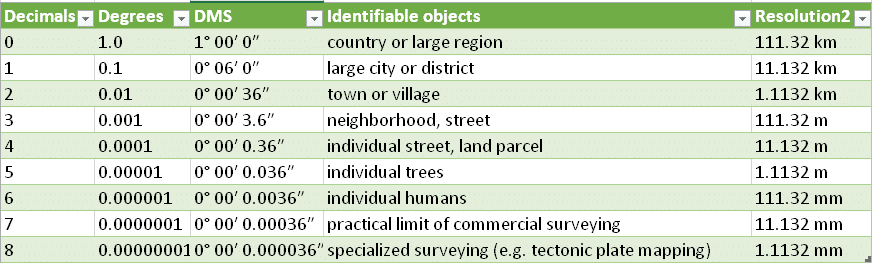# Power BI Lat Long Converter

Power BI and Power Map support mapping your own data using Latitude and Longitudes. Lat-longs are available in various formats. Power BI supports only decimal format. Unfortunately lot of data still comes with the DMS format Lat-Longs. I created a simple tool: Power BI Lat Long Converter. Use it do perform bulk conversions quickly.

## Power BI Lat Long Converter

Data is used in various ways in the context of Power BI. Sometimes, we just want to use Excel. Or we can use Power Query. In addition, the stand-alone Power BI Desktop tool can also be used for data management and clean-up.

In this article I am providing you with all three methods. Simple Excel formulas, Power Query or Power BI Desktop.

## Input format

The DMS format specifies the direction and the angle in Degrees Minutes and Seconds.

Latitude is vertical – therefore it has North or South direction indicator. Longitude is vertical – it has East or West components.

41°25’01″N and 120°58’57″W

First number is Degrees (54), second one is Minutes (25) and third number is Seconds (01). Performing calculations based upon this notation requires lot of string manipulation. Sometimes the N or S is written before the numbers. Sometimes the symbols for degrees, minutes and seconds are missing. Therefore, it is best to split the data into its individual components before conversion.

This can be done using Text to Columns in Excel or using Power Query split functions. Finally, try to get the raw data in the format shown below. First four columns are the Latitude data. Column 5 to 8 are Longitude data. Make sure that there are no empty cells. Put zero instead of empty cells. This matter for Power BI based calculations. Empty cells are considered as null by Power BI. Therefore, it will product null  output.## Conversion formula

Power BI Lat Long Converter uses the following formulas to convert the DMS coordinates to Decimal.

The degrees value remains as it is. The minutes and second values need to be converted to the decimal portion of the number. The direction decides whether the number is positive or negative.

Minutes are divided by 60 and seconds by 3600. Add them up to the degrees value and we have the decimal version.

Download LatLongConverter.zip Unzip it. It contains two files. The Excel file shows formula based and Power Query based conversion. The PBIX file shows Power BI Desktop based conversion.

### Excel formulas for conversion

The attached Excel file contains multiple sheets. DMS to Decimal sheet has data that is already split as shown above. It has two more columns LatExcel and LongExcel. Learn the formulas given there. This data is a table. You can delete all except the first row and append your own data to autofill all the formulas and perform bulk conversion instantly. Remember to delete the first row after bulk conversion is completed.

### Power Query based conversion

Power Query is a great new data import and clean-up tool. You can install it on top of Excel 2010 and 2013. It comes built-in with Excel 2016. In 2006 it is under the Data tab – Get and Transform area.

In this sample file, I have used the same data from Excel and imported it into Power Query. I have added two more columns LatPQ and LongPQ which show exactly the same results as the LatExcel and LongExcel columns. I just wanted to show the same logic being applied in Power Query.

You can edit the query and learn the syntax. Power Query is also using the same formula as Excel, but its syntax is a little different.

### Power BI Desktop File

Nowadays, many of my customers are using Power BI Desktop instead of Excel based Power BI. For their benefit, I have given the same content in PBIX format.  Choose Edit Query to view the formulas.

The data can now be converted to a report using the Map visualization. Drag Drop LatPQ and LongPQ into Latitude and Longitude areas in the Fields area.## Using with Power Map

For Power Map, you need to use the Lat Long fields and choose Latitude and Longitude from the drop down. Power Map will then plot the locations instantly.## Power BI Lat Long Converter – Precision

The decimal format can have many decimal places. Depending upon the object in question, the number of decimals matter. Here is a simple table to illustrate the importance of how many decimals you need.  Source Wikipedia.